How Cheenta works to ensure student success?
Explore the Back-Story

# Trigonometry & natural numbers | PRMO 2017 | Question 11Try this beautiful problem from the Pre-RMO, 2017 based on Trigonometry & natural numbers.

## Trigonometry & natural numbers - PRMO 2017

Let f(x) =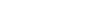for all real x, find the least natural number x such that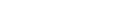for all real x.

• is 107
• is 60
• is 840
• cannot be determined from the given information

### Key Concepts

Trigonometry

Least natural number

Functions

PRMO, 2017, Question 11

Plane Trigonometry by Loney

## Try with Hints

here f(x) =period of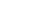is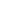period of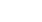is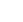Lcm=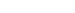.

## Subscribe to Cheenta at Youtube

Try this beautiful problem from the Pre-RMO, 2017 based on Trigonometry & natural numbers.

## Trigonometry & natural numbers - PRMO 2017

Let f(x) =for all real x, find the least natural number x such thatfor all real x.

• is 107
• is 60
• is 840
• cannot be determined from the given information

### Key Concepts

Trigonometry

Least natural number

Functions

PRMO, 2017, Question 11

Plane Trigonometry by Loney

## Try with Hints

here f(x) =period ofisperiod ofisLcm=.

## Subscribe to Cheenta at Youtube

This site uses Akismet to reduce spam. Learn how your comment data is processed.

### Knowledge Partner